# An Overview of Density Estimation

Density estimation is estimating the probability density function of the population from the sample. This post examines and compares a number of approaches to density estimation.

By Ajit Samudrala, Data Scientist at Symantec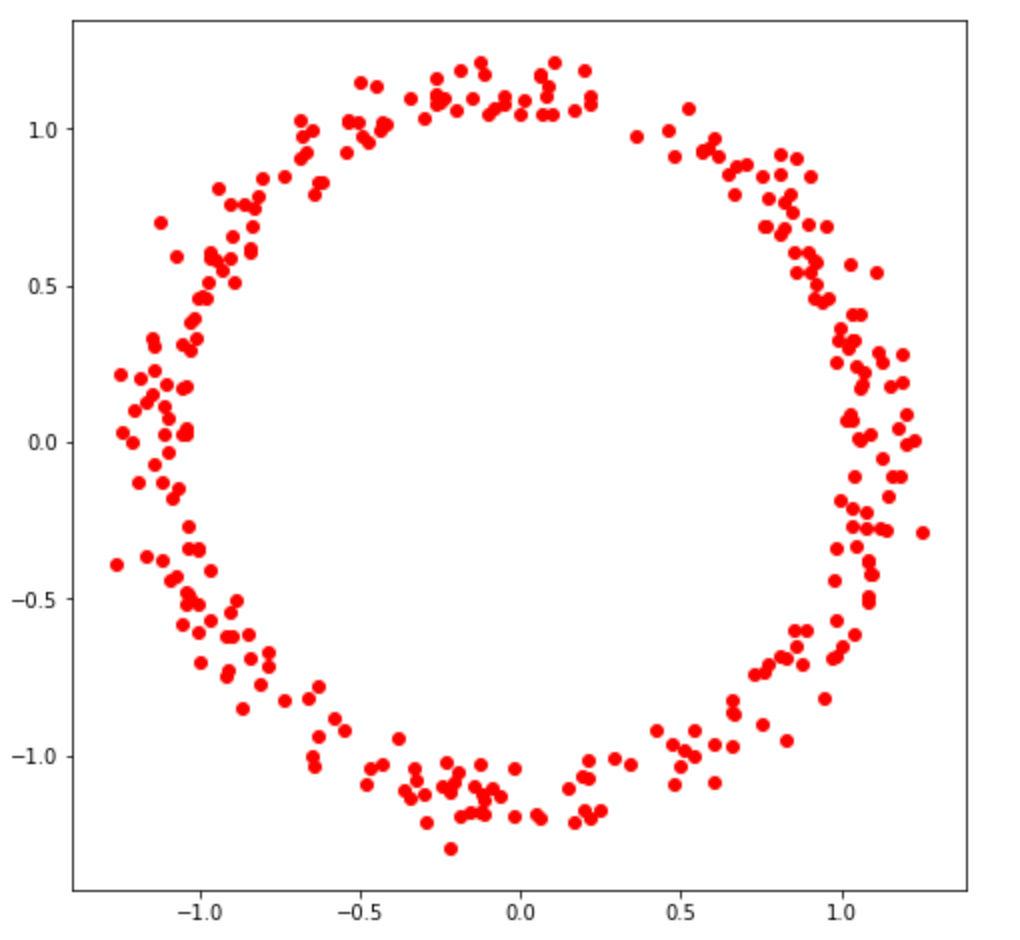Statistics revolve around making estimations about the population from a sample. Density estimation is estimating the probability density function of the population from the sample.

### Terminology

Estimator: Function of data that approximates a parameter of interest. An estimator is a random variable as it is a function of a random sample.

Consistent Estimator: Any estimator that converges to the true parameter of interest as the sample size tends to infinity is called a consistent estimator. The sample mean is a consistent estimatorUnbiased Estimator: Any estimator whose expected value is equal to the true parameter of interest is called unbiased estimator. The sample mean is an unbiased estimator.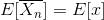Parametric Methods: We assume the population follows a certain distribution and try to estimate its parameters from the available data.

Non-parametric Methods: No assumptions are made on the population distribution. While parametric methods only involve estimating few parameters, non-parametric methods try to estimate density on the entire sample space.

Explicit Density Estimation: Estimates the true pdf or cdf over the sample space.

Implicit Density Estimation: Doesn’t produce explicit densities but generates a function that can draw samples from the true distribution.

### Maximum Likelihood Estimation (MLE)

MLE is a parametric method that maximizes the likelihood or log-likelihood function, which is a function of parameters. MLE is a random variable as it is calculated on a random sample. MLE is a consistent estimator and under certain conditions, it asymptotically converges to a normal distribution with true parameter as mean and variance equal to the inverse of the Fisher information matrix.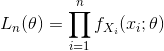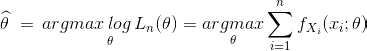For example, if we assume our population follows a normal distribution, which is parametrized by mean and variance, the MLE is the sample mean and rescaled sample variance.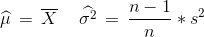Consistency of MLE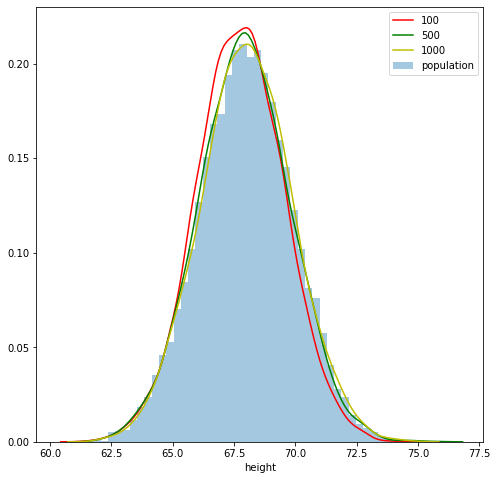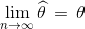As aforementioned MLE is a consistent estimator i.e., as the sample size increases the MLE approaches true parameter, which is demonstrated in the above figure.

### Maximum-a-Posteriori Estimation (MAP)

MAP takes our prior belief on true parameter into account, while calculating its posterior likelihood. If our prior belief is uniform on the parameter space, MLE is equal to MAP.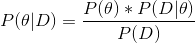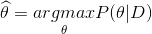### Method of Moments (MOM)

According to the Law of Large Numbers (LLN), the average converges to the expectation as the sample size tends to infinity. Using this law, the population moments which are a function of parameters are set equal to sample moments to solve for the parameters. For a normal distribution, both MLE and MOM produce sample mean as an estimate to the population mean.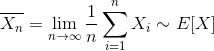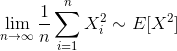### Empirical Cumulative Distribution Function (ECDF)

Another way to characterize data generating distribution a.k.a population distribution is estimating its cdf instead of pdf. ECDF is a consistent estimator, unbiased estimator and non-parametric.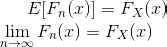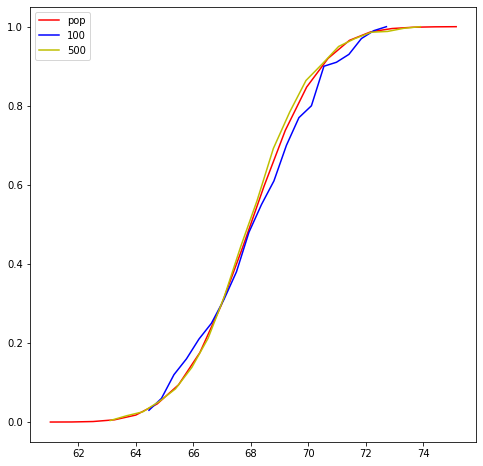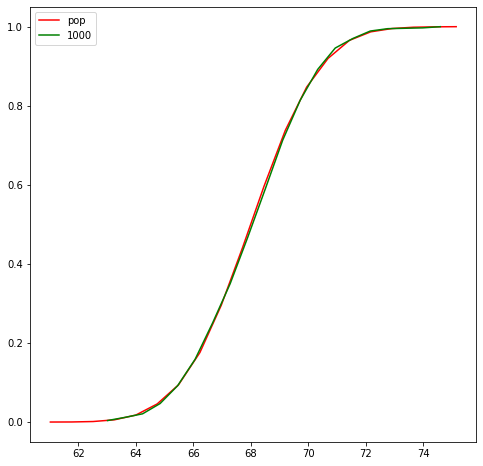As the sample size increases, the ecdf becomes close to the true cdf.

### Kernel Density Estimation (KDE)

KDE is a non-parametric method to estimate pdf of data generating distribution. KDE allocates high density to certain `x` if sample data has many datapoints around it. A datapoint’s contribution to certain `x` depends on its distance to `x` and bandwidth. As the sample size increases, KDE approximation under certain conditions approaches true pdf.Kernel function should always produce a value greater than or equal to zero and it should integrate to 1 over the sample space. Some popular kernels are uniform, gaussian, biweight, etc.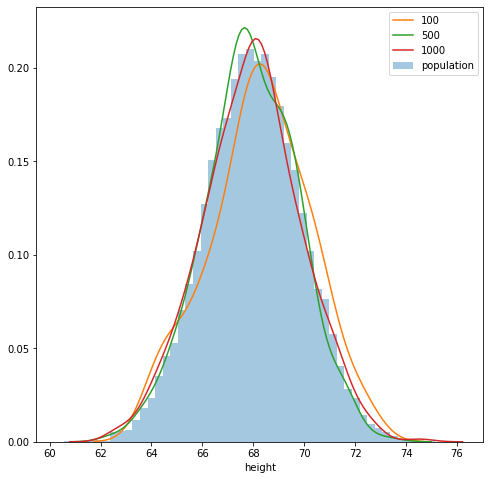bandwidth: Scott, Kernel: Gaussian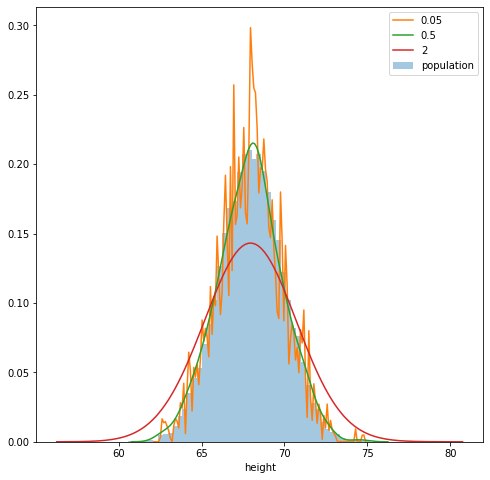effect of bandwidth

As large bandwidths, it overestimates the density at points with fewer data points around them thus over smoothening the curve. On the other hand, if the bandwidth is very small it produces spurious fluctuations and overfits to the data.

### Q-Q Plot

Plots the quantiles of two distributions against each other, typically against a theoretical distribution with know parameters like a standard normal distribution. If the sample is generated from a data distribution that is identical to a theoretical distribution, the q-q plot looks like a straight line.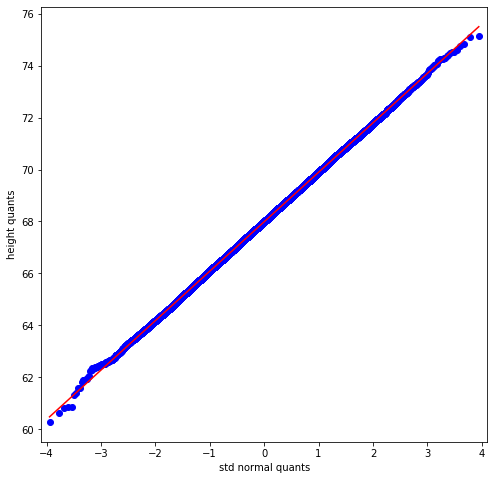Though Q-Q plot doesn’t estimate true pdf, it is a quick and easy way to check our assumptions about true pdf.

### GAN

A GAN takes a random sample from a latent distribution as input and maps it to the data space. The task of training is to learn a deterministic function that can efficiently capture the dependencies and patterns in the data so that the mapped point resembles a sample generated from the data distribution. Below I have generated 300 random samples from `Isortropic Bivariate Gaussian` distribution.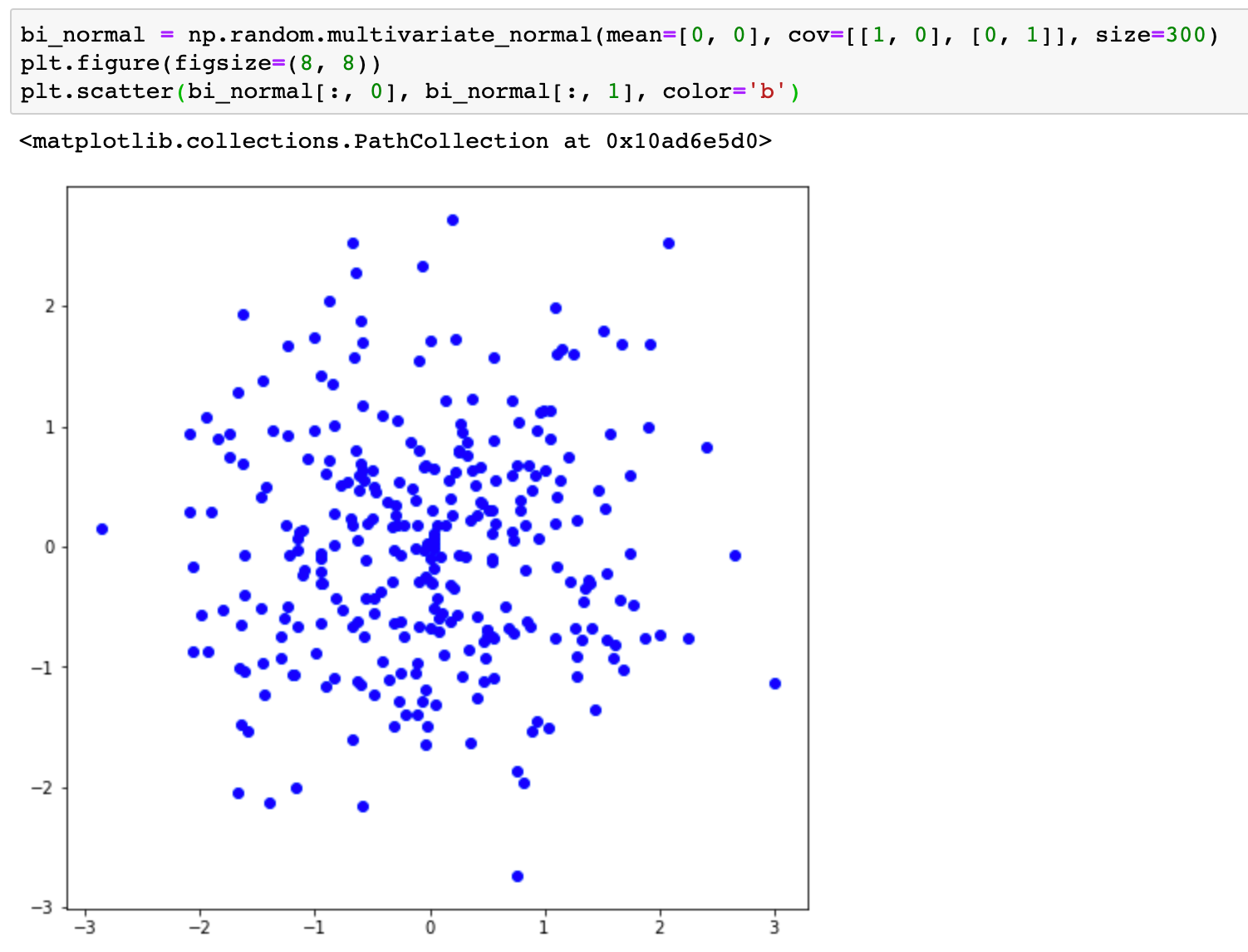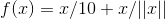When passed through the above function, the samples form a ring. In GAN, this function is essentially the generator network.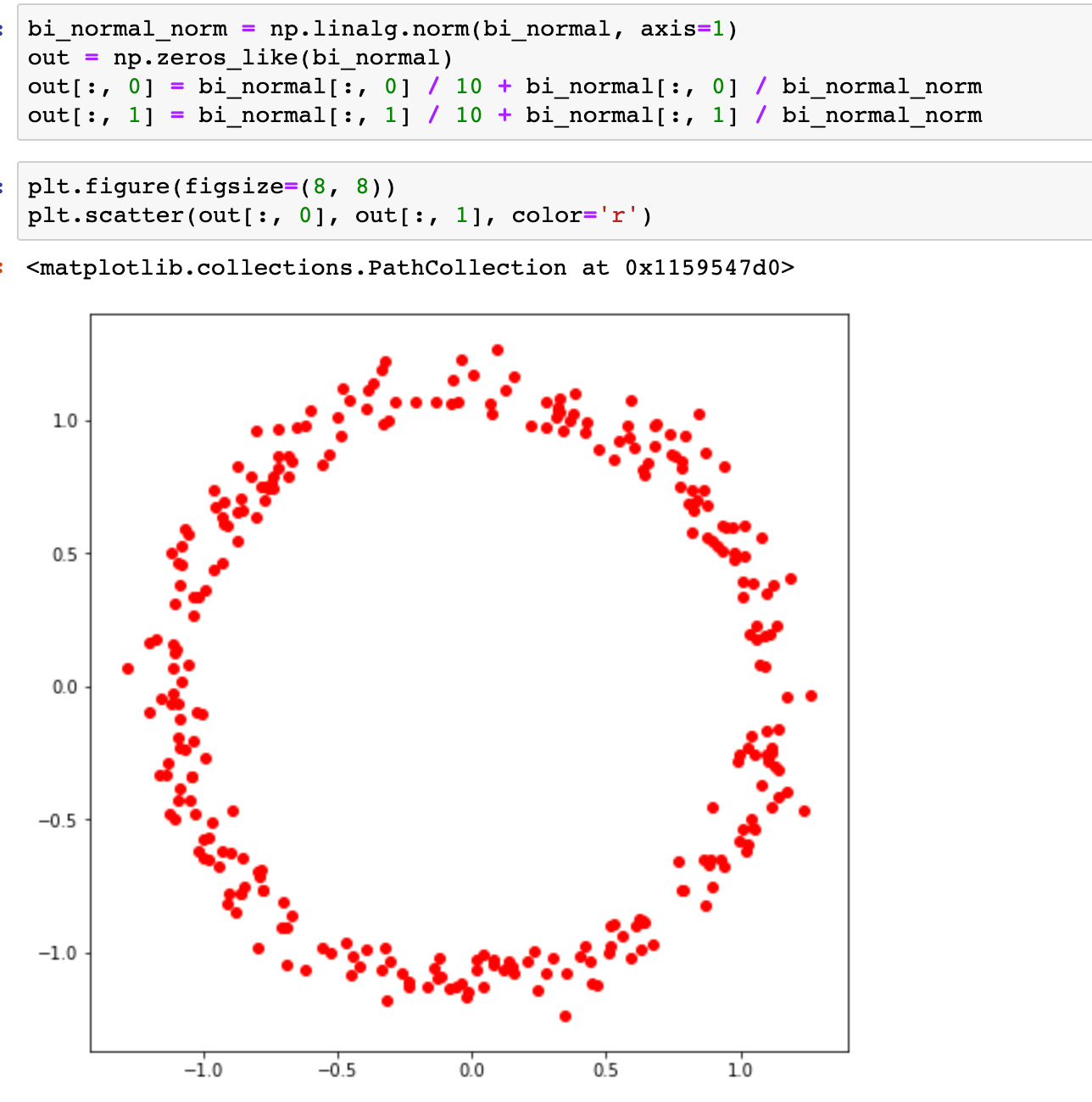The above example demonstrates that there could be a high capacity function that may be able to model data distribution of high dimensional data like images. Neural networks are out best bet as they are universal functional approximators. Hence, deep neural networks are used while modeling data distribution of images.

I have just introduced the topics in the article. Read more about them to strengthen your understanding of them. Find the complete notebook here.

Bio: Ajit Samudrala is a Data Scientist at Symantec. He enjoys learning and implementing machine learning.

Original. Reposted with permission.

Related: